# Determining ceramic particle sizes suspended in a fluid

• Tiberious
In summary, the experiment showed that the particles descended at a range of speeds, with the largest particles being 5 millimeters in diameter. The density of the material was 3800 kilograms per meter squared, and the density and viscosity of the fluid at room temperature were 1632 kilograms per meter squared and 0.00972 Pas respectively. The equation for the particles' descent was rearranged to make it easier to read, and the units for all the variables were included.f

## Homework Statement

(I) An experiment to determine ceramic particle sizes showed that the rate of descent when suspended in a fluid ranged from 1.2 × 10–6 m s–1 to 5 × 10–6 m s–1.

The density of the material was 3800 kg m–3 and the density and viscosity of the fluid at room temperature were 1632 kg m–3 and 0.00972 Pas respectively. Calculate the range of sizes of the particles.

## Homework Equations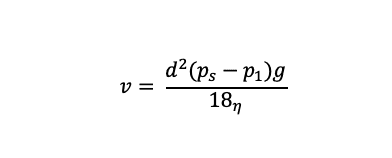## The Attempt at a Solution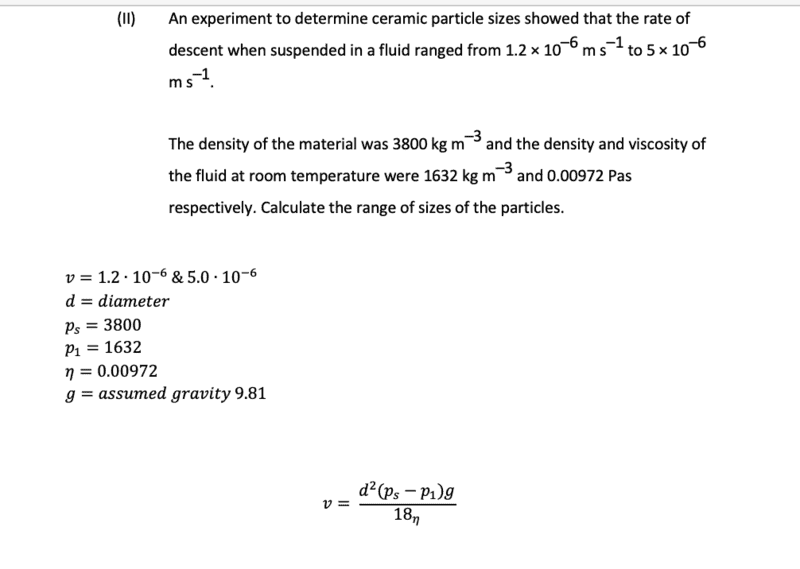[/B]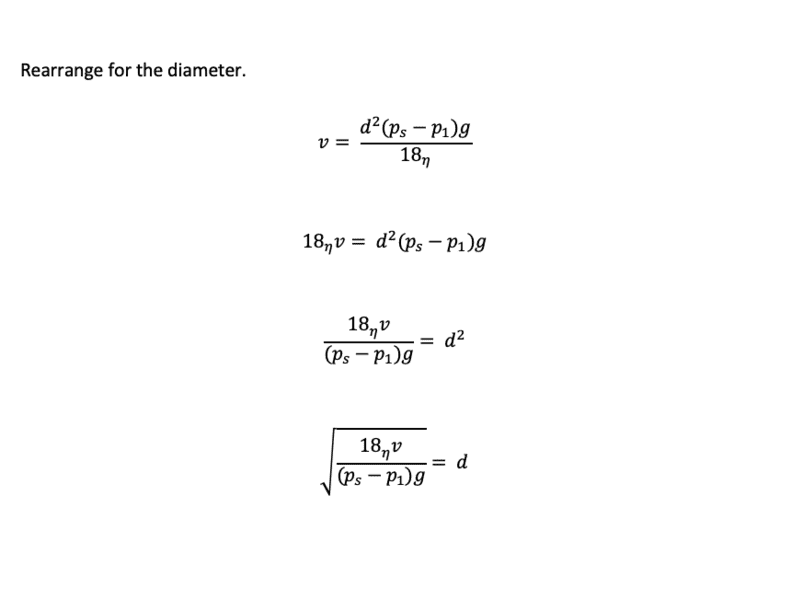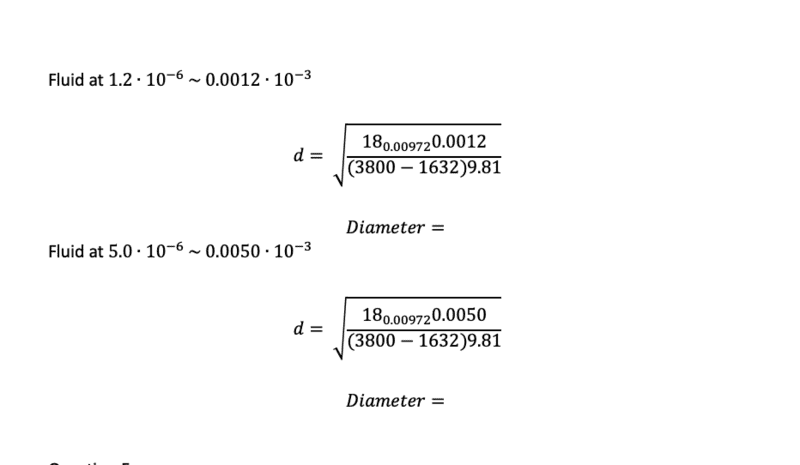I've recently completed the above, though the diameters have not yet been calculated. Does the rearrangement of the original equation look correct ?

#### Attachments

Tangential, we used to measure particle size of an antacid dispersal and an iron supplement by an electrical method related to blood-cell counting.

IIRC, a representative dilution was trickled through a submerged capillary nozzle, and the variations in conductivity between carrier and carrier + particle cleverly logged by multiple parallel 'box-car' circuits. Getting it to work reliably was a bit of an art, to say the least. My understanding was that its digital replacement was rather more user-friendly...

Thank-you, any ideas as towards the rearrangement of the formula ?

Just from an Algebraic standpoint, the re-arrangement looks good to me!

However I wonder about the particle sizes. In particular their are no units-of-measure in the problem statement. Are the units consistent in your numerical representation?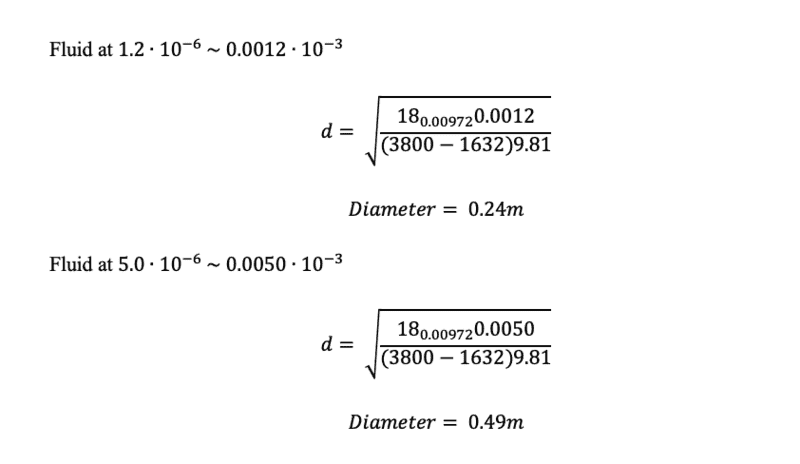As the question was in microns and the material in milli, I've converted to milli.

The above should read mm. Apologies for that.

#### Attachments

You should keep units, otherwise it is difficult to catch conversion errors.
The denominator still has (omitted) meters everywhere, for example.

A bit tangential to the problem, but it can make googling for additional materials easier: this is an application of Stokes' law, common in measurement of dynamic viscosity (η in your equations).

•Tom.G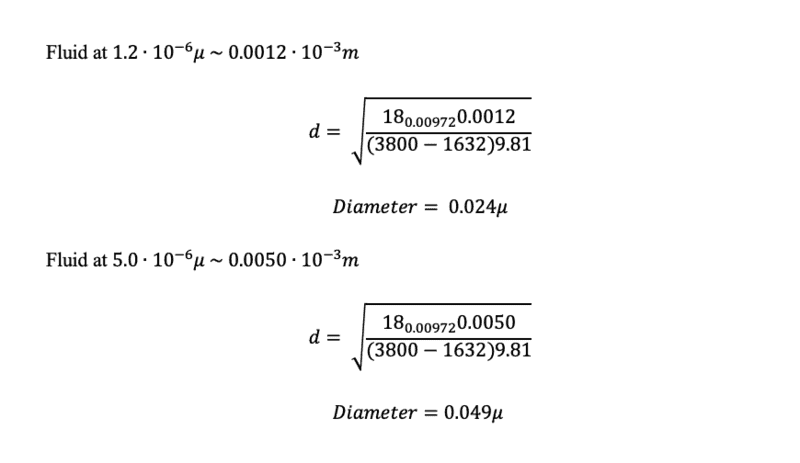Hiya all really appreciate the feedback, I've added some units to the equation. Does the above seem accurate ? Any obvious omissions ?

#### Attachments

$$d = \sqrt{\frac{18_{0.00972}0.0050}{(3800-1632)9.81}}$$
I don't see any units there, the correct units would need conversion to cancel, and I don't understand why ##\eta## is written as subscript.

You can use LaTeX here in forum posts by the way, just put it in double # (inline) or double \$ (separate line).

and I don't understand why ##\eta## is written as subscript.

Perhaps because ##180.009720.0050## was unreadable (as apposed to ##18\times0.00972\times0.0050##)

Apologies the subscript was an error when I read the original equation, it was actually to the base of that figure. I've corrected in the attached and included the relevant units within the equation.

Latex is something I will certainly need to learn.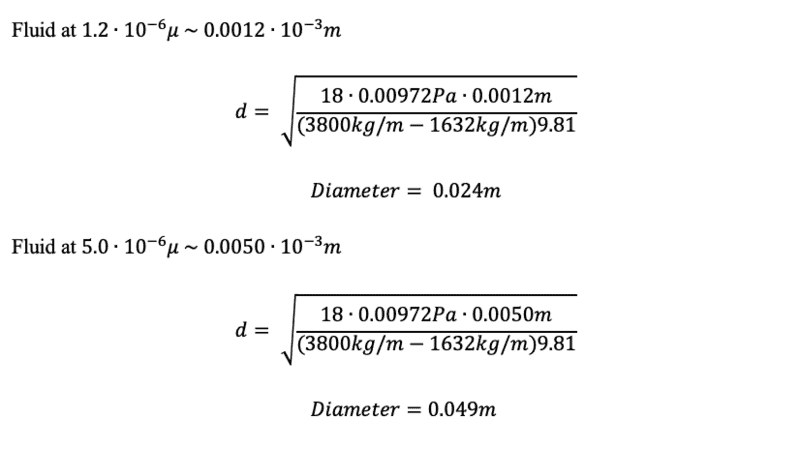#### Attachments

As the question was in microns
View attachment 237765
No, the question as posted is all in SI (MKS) units The values of the speeds merely happen to be in the micron/sec range. So there is no need to do any conversions.

In the attachment in post #11, each μ should be m.
And having obtained 0.0012 10-3m, what happened to the 10-3, and what happened to the s-1?

Edit: and what did you think of the 5cm diameter answer you got?

Last edited:
And having obtained 0.0012 10-3m, what happened to the 10-3, and what happened to the s-1?
And the m3 of the density, and the units of g and the viscosity. Out of 5 factors in the expression only the dimensionless 18 is without error.

@Tiberious: Get your units right. Physics does not work without units.

@haruspex, larger than expected, I see my error converting at the start and will arrange the units accordingly in my answer. Appreciate the assistance.